# 求最小生成树的算法和求最短路径的算法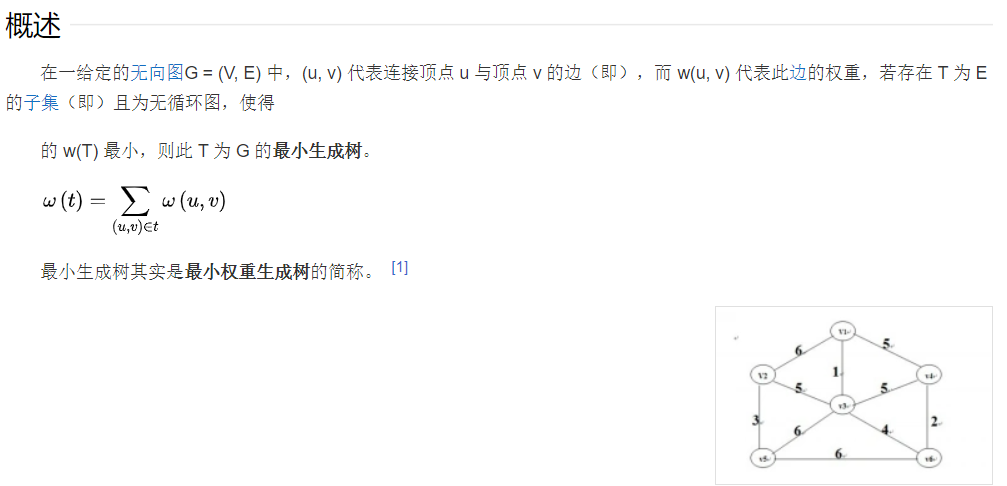## 最小生成树算法

### kruskal算法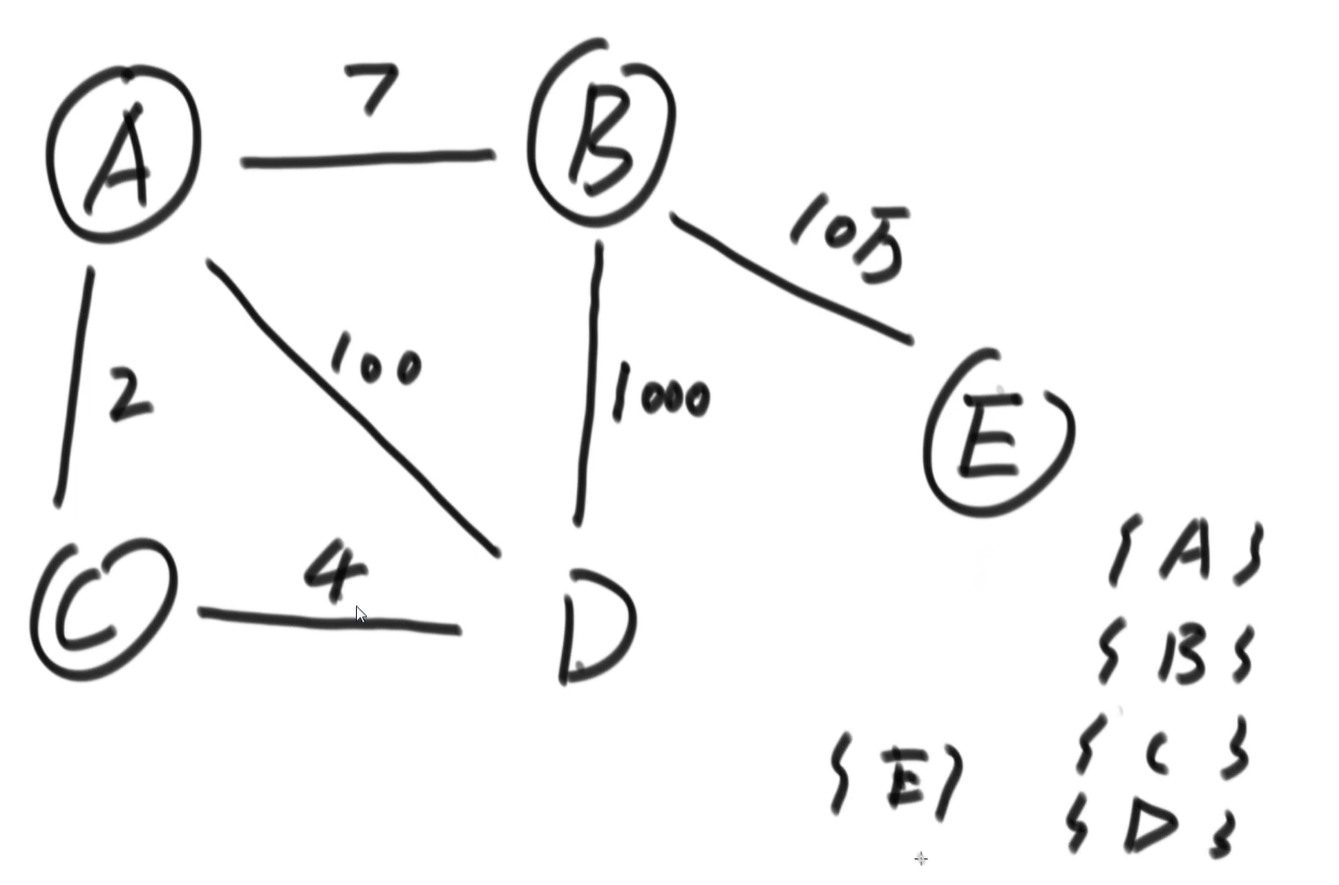``````public static class MySets{

public HashMap<Node, List<Node>> setMap;

public MySets(List<Node> nodes) {
for (Node cur : nodes) {
List<Node> set = new ArrayList<Node>();
setMap.put(cur, set);
}
}

public boolean isSameSet(Node from, Node to) {
List<Node> fromSet = setMap.get(from);
List<Node> toSet = setMap.get(to);
// 判断toSet和fromSet的地址是否相同
return fromSet == toSet;
}

public void union(Node from, Node to) {
List<Node> fromSet = setMap.get(from);
List<Node> toSet = setMap.get(to);
// 把to中所有的node都加到form中去，将to中所有的node指向fromSet
for (Node toNode : toSet) {
setMap.put(toNode, fromSet);
}
}
}

public static Set<Edge> kruskalMST(Graph graph) {
// graph.nodes.values()方法返回的是装有图中所有节点的List集合
Mysets mysets = new Mysets(graph.nodes.values());
// EdgeComparator()比较的是优先小边
PriorityQueue<Edge> priorityQueue = new PriorityQueue<>(new EdgeComparator());
for (Edge edge : graph.edges) {
}
Set<Edge> result = new HashSet<>();
while (!priorityQueue.isEmpty()) {
Edge edge = priorityQueue.poll();
if (!mysets.isSameSet(edge.from, edge.to)) {
mysets.union(edge.from, edge.to);
}
}
return result;
}
``````

### prime算法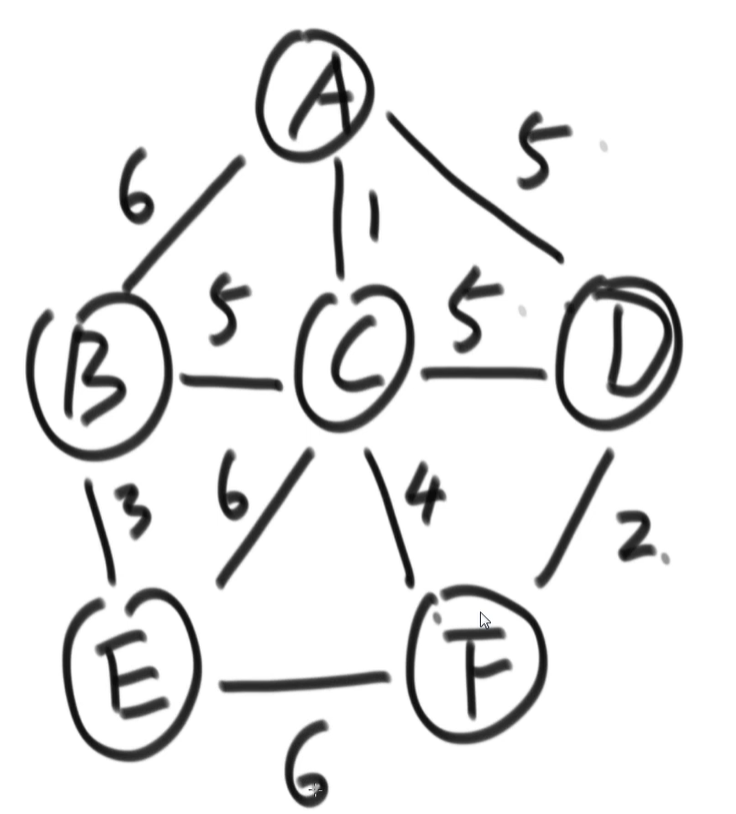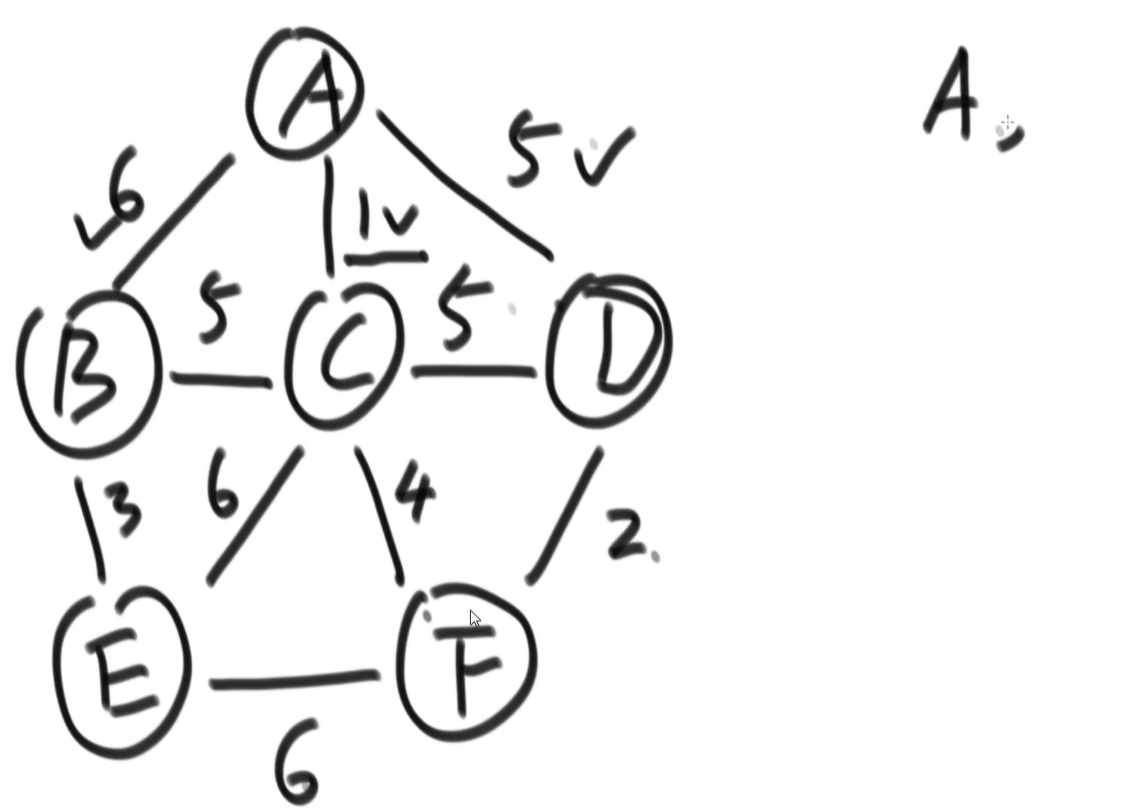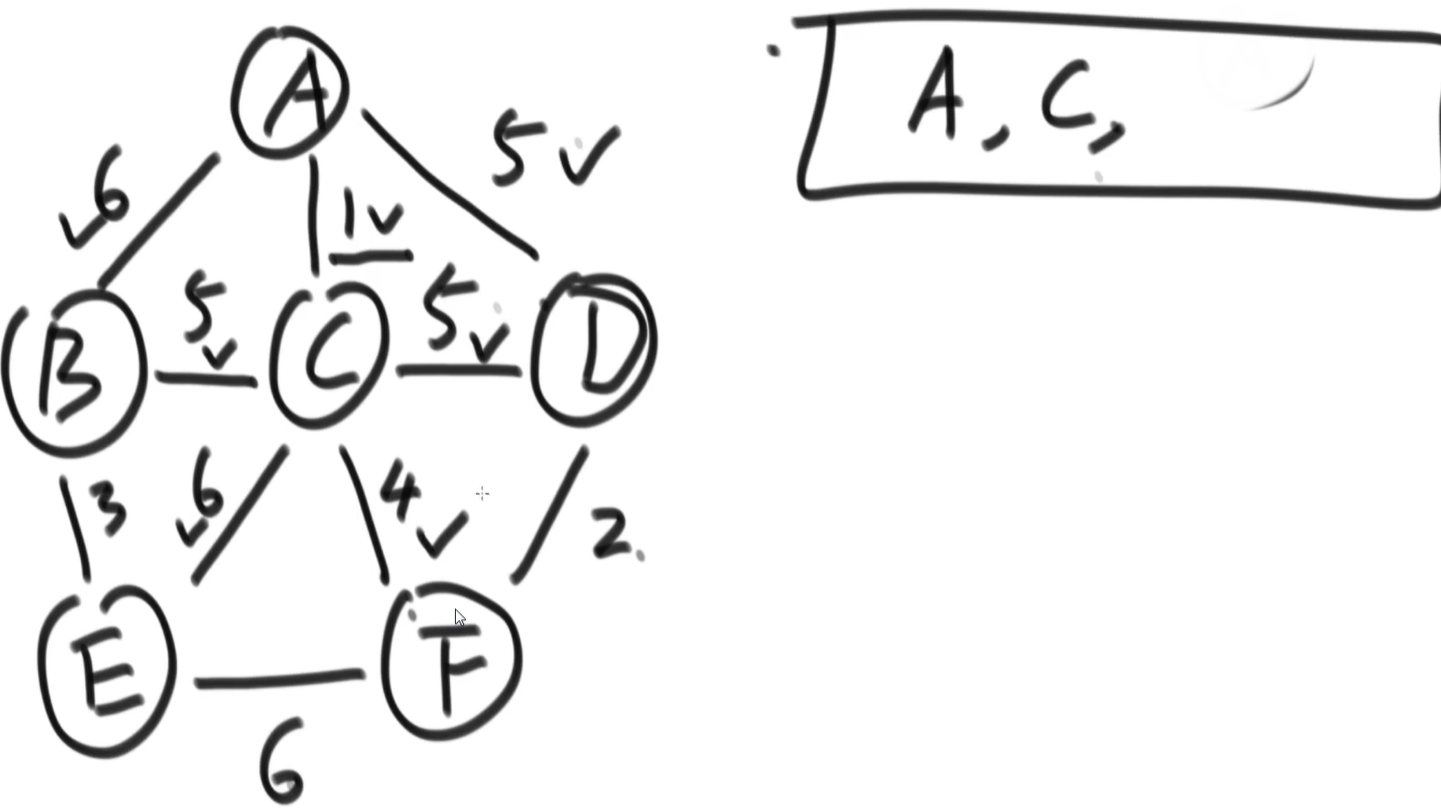``````public static Set<Edge> primMst(Graph graph) {

// 解锁的边进入小根堆
PriorityQueue<Edge> priorityQueue = new PriorityQueue<>(new EdgeComparator);

// 存放节点的set
HashSet<Node> set = new HashSet<>();

// 依次挑选的边在result中
Set<Edge> result = new HashSet<>();

// 这个foreach循环是考虑森林的问题，即不连通的多张图，都要把每张图的最小生成树加入result中，如果是一个图不需要这个foreach
for (Node node : graph.nodes.values()) {

// 随便挑一个点
// node是开始点
if (!set.contains(node)) {
for (Edge edge : node.edges) {
// 由一个点，解锁所有相连的边
}
while (!priorityQueue.isEmpty()) {
// 弹出已经解锁的边中，最小的边
Edge edge = priorityQueue.poll();
// 可能的一个新的点
Node toNode = edge.to;
// 如果set中不含有这个toNode节点，那么用这个点
if (!set.contains(toNode)) {
for (Edge nextEdge : toNode.edges) {
}
}
}
}

}
return result;
}
``````

### 细节

k算法由于将权值排列后放入小根堆中，每次弹出的边不具有连续性，所以会存在节点跳跃的情况。

p算法由于是从点出发，每次都是选择一个点加入set，所以不会存在上述情况。但是由于我们也用了小根堆，每次加入set的新节点要重复《将这个节点的所有边加入小根堆》，会导致上次选择过的边再次加入小根堆。即使每次小根堆弹出后会判断连接的节点是否是新的，不会印象最终结果，但也会消耗更多时间。

## 最短路径算法

### Dijkstra算法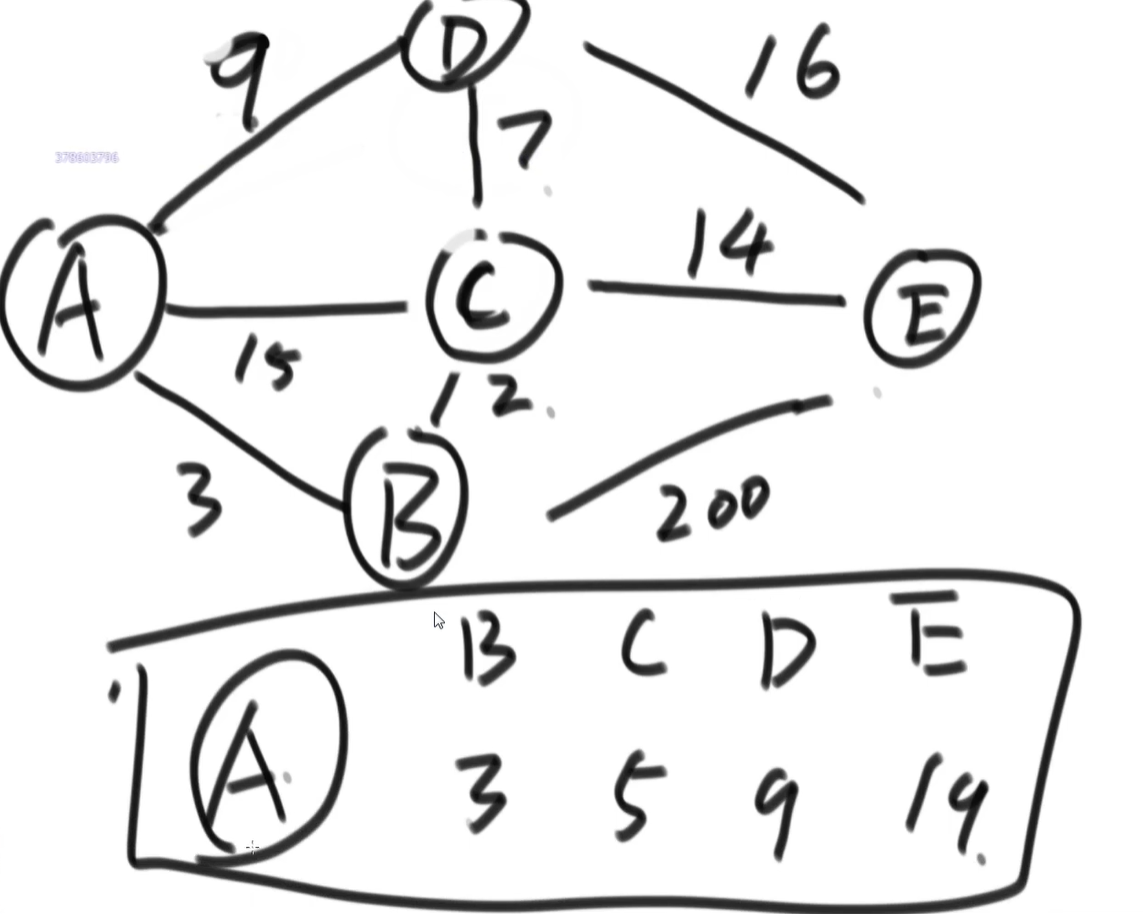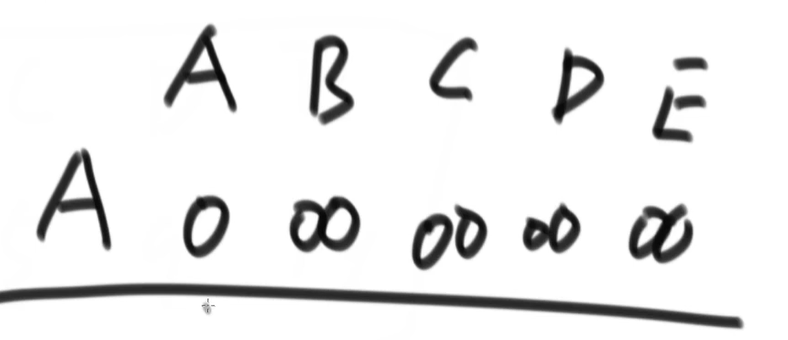《锁死A》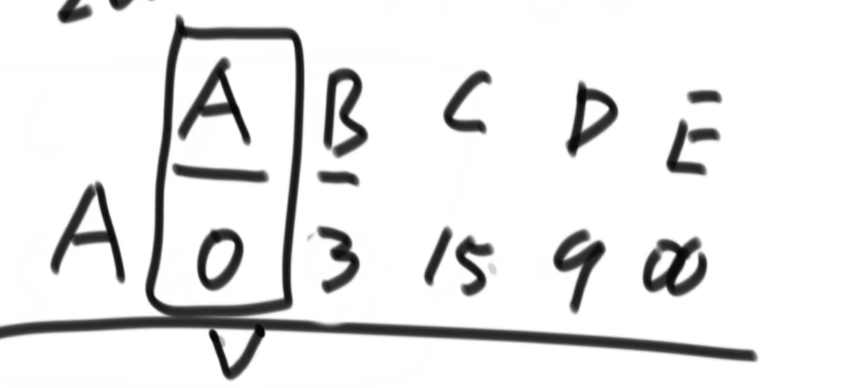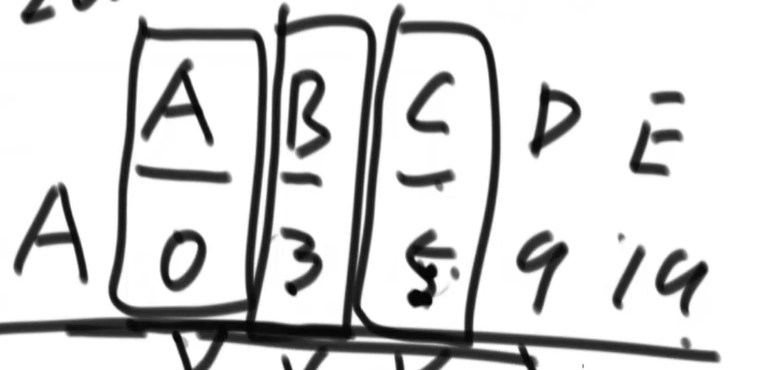``````public static HashMap<Node, Integer> dijkstra1(Node head) {
HashMap<Node, Integer> distanceMap = new HashMap<>();
// 已经求过的距离的节点,存在selectedNodes中,以后再也不碰
HashSet<Node> selectedNodes = new HashSet<>();
// 将头节点的连接都记录下来
Node minNode = getMinDistanceAndUnselectedNode(distanceMap, selectedNodes);
while (minNode != null) {
int distance = distanceMap.get(minNode);
for (Edge egde : minNode.edges) {
Node toNode = edge.to;
if (!distanceMap.containsKey(toNode)) {
distanceMap.put(toNode, distance + edge.weigth);
}else {
distanceMap.put(edge.to, Math.min(distanceMap.get(toNode), distance + edge.weight));
}
}
minNode =getMinDistanceAndUnselectedNode(distanceMap, selectedNodes);
}
return distanceMap;
}

public static Node getMinDistanceAndUnselectedNode(HashMap<Node, Integer> distanceMap, HashSet<Node> touchedNodes) {
Node minNode = null;
int minDistance = Integer.MAX_VALUE;
for (Entry<Node, Integer> entry : distanceMap.entrySet()) {
Node node = entry.getKey();
if (!touchedNodes.contains(node) && distance < minDistance) {
minNode = node;
minDistance = distance;
}
}
return minNode;
}
``````